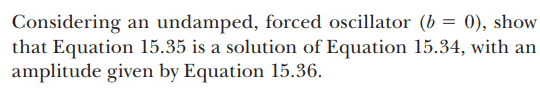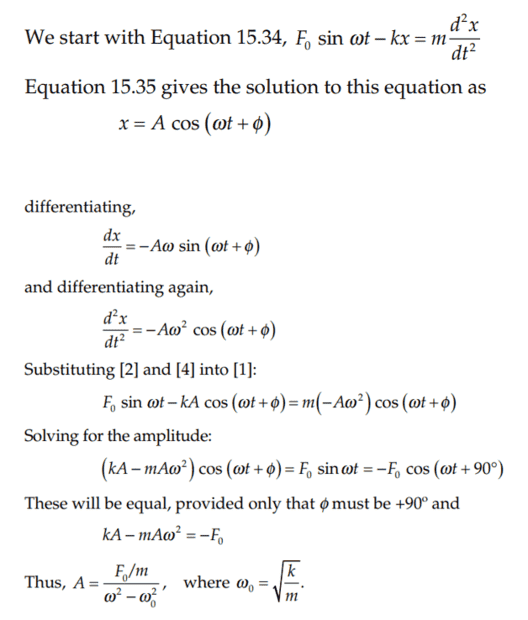# Undamped forced oscillator

Callumnc1
Homework Statement:
Relevant Equations:
For this problem,The solution is,However, can someone please explain how this is showing equation 15.35 as a solution of equation 15.34? I though both sides should be equal without assuming that ##\phi = 90##

Also why are they allowed to assume ##\phi = 90##?

Many thanks!

Homework Helper
Gold Member
2022 Award
Given 15.34, we want to see whether an equation of the form 15.35 can be a solution if we plug in suitable values for ##A, \omega, \phi##.
To check this, we use 15.35 to substitute for x in 15.34.
It turns out that 15.34 is satisfied by 15.35 provided ##\phi=\pi/2+2n\pi## and ##A=\frac{F_0}{m\omega^2-k}##.

•Callumnc1
Callumnc1
Given 15.34, we want to see whether an equation of the form 15.35 can be a solution if we plug in suitable values for ##A, \omega, \phi##.
To check this, we use 15.35 to substitute for x in 15.34.
It turns out that 15.34 is satisfied by 15.35 provided ##\phi=\pi/2+2n\pi## and ##A=\frac{F_0}{m\omega^2-k}##.

Homework Helper
2022 Award
and any solution to the unforced (or homogeneous or ##F_0=0##) system can be added to produce another solution as dictated by initial conditions.

•Callumnc1
Callumnc1
and any solution to the unforced (or homogeneous or ##F_0=0##) system can be added to produce another solution as dictated by initial conditions.
Thank you @hutchphd , that is good to know!

•hutchphd
Gold Member
I though both sides should be equal without assuming that ϕ=90

Also why are they allowed to assume ϕ=90?
Φ and A were not defined initially. They were testing a function to see what would be required for it it satisfy the original equation. Then they derived what Φ and A had to be for that form to be correct.

•Callumnc1
Callumnc1
Φ and A were not defined initially. They were testing a function to see what would be required for it it satisfy the original equation. Then they derived what Φ and A had to be for that form to be correct.
Oh ok thank you @DaveE that makes more sense now!